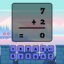Hlavní navigace

#TypeMaths 1.0

TypeMaths app is an exciting entertainment app. It is a fun and entertainment learning app for preschool children and toddlers including those with autism. It will learn simple mathematical equation solving into this app.

How to play?
Player can choose operation from the dashboard and then try to solve all equation of that selected equation.
Player have one mathematical equation of selected operation and also have custom keyboard for type the answer of given equation so player can solve given equation and type the answer of that equation by custom keyboard and press next for new equation.
It have functionality of speak total equation as help to solve that equation.
It also display right and wrong answer count of current level.

Try it free for understanding mathematical equation solving with batter way of learning.

## Celkové hodnocení

Průměr hodnocení
3

Pro hodnocení programu se prosím nejprve

## Souhrnné informace o TypeMaths

1.0

ne

29,85 MB

• Angličtina
• #### Staženo

0× celkem
0× tento měsíc

15. 5. 2020

## Něco jsme propásli?

Dejte nám vědět. Upozornit redakci Stahuj
Velice děkujeme za Vaše podněty